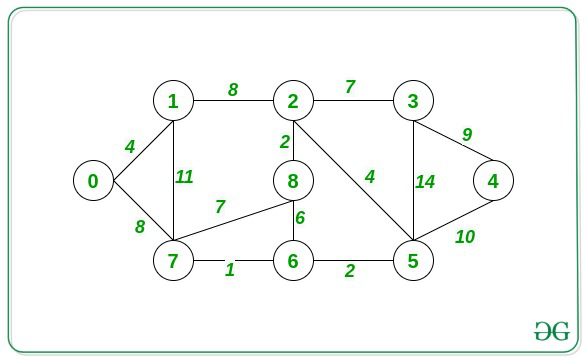# Shortest distance between two nodes in Graph by reducing weight of an edge by half

• Difficulty Level : Basic
• Last Updated : 06 Sep, 2021

Given a weighted undirected graph consisting of N nodes and M edges, the task is to find the shortest distance between two nodes A and B by reducing the weight of one edge by half.

Examples:

Input: A = 0, B = 2, Below is the graphOutput: 8
Explaination:
After reducing the weight of the edge connecting 1 and 2 by half modifies its new weight to 4. Now, the shortest distance to reach 2 from 0 through the path 0 -> 1 -> 2 is (4 + 4) = 8.
Therefore, print 8.

Approach: The given problem can be solved by maintaining two arrays, the shortest distance array taking source node as A which will store the shortest distance of all nodes from A, and similarly the shortest distance array taking source node as B. These arrays can be calculated using Dijkstra’s algorithm. Follow the below steps to solve the above problem:

• Use Dijkstra’s algorithm to store the shortest distance of each node from A into an array disA[].
• Use Dijkstra’s algorithm to store the shortest distance of each node from B into an array disB[].
• Suppose edgei = {ui, vi, wti} i.e., edgei connects node ui to vi and has a weight of wti.
• Now, iterate over all edges and for every edge keep track of the function as:

f(edgei) = min( disA[ui] + disB[vi], disA[vi] + disB[ui]) + (wti/2).

• The above relation gives the minimum value of f(edge), which is the resultant shortest distance.

Below is the implementation of the above approach:

## C++14

 `// C++ program for the above approach` `#include ``using` `namespace` `std;` `// Stores the input Graph``vector > graph;` `// Stores edges of input Graph``vector > edges;` `// Function to input Edges``void` `add_edge(``int` `u, ``int` `v, ``int` `w)``{``    ``graph[u].push_back({ v, w });``    ``graph[v].push_back({ u, w });``    ``edges.push_back({ u, v, w });``}` `// Function to find the shortest distance``// to each node from the src node using``// Dijkstra’s Algorithm``vector<``int``> dijsktras(``int` `src, ``int` `N)``{``    ``// Stores the shortest distance of``    ``// each node form src node``    ``vector<``int``> dis(N, INT_MAX);` `    ``vector<``bool``> vis(N, ``false``);` `    ``// Stores the node and current``    ``// minimum distance in a heap``    ``priority_queue,``                   ``vector >,``                   ``greater > >``        ``pq;``    ``pq.push({ 0, src });``    ``dis[src] = 0;` `    ``// BFS for single source shortest``    ``// path algorithm``    ``while` `(!pq.empty()) {` `        ``// Top of the PQ``        ``auto` `cur = pq.top();``        ``pq.pop();` `        ``// Store the node and weight``        ``int` `node = cur.second;``        ``int` `weight = cur.first;` `        ``// If node is already visited``        ``if` `(vis[node])``            ``continue``;``        ``vis[node] = ``true``;` `        ``// Traverse the adjacency list``        ``// of the node``        ``for` `(``auto` `child : graph[node]) {` `            ``// If the distance obtained``            ``// from parent is less than``            ``// the current minimum``            ``// distance stored for child``            ``if` `(dis[child.first]``                ``> child.second + weight) {``                ``dis[child.first] = weight``                                   ``+ child.second;` `                ``// Push the next pair``                ``// in the PQ``                ``pq.push({ dis[child.first],``                          ``child.first });``            ``}``        ``}``    ``}` `    ``// Return the maximum distance``    ``return` `dis;``}` `// Function to find shortest distance``// between two nodes by reducing any``// one weight of an edge by half``int` `shortestDistance(``int` `N, ``int` `M,``                     ``int` `A, ``int` `B)``{``    ``// Stores the the shortest distance``    ``// of each node from A``    ``vector<``int``> disA = dijsktras(A, N);` `    ``// Stores the the shortest distance``    ``// of each node from B``    ``vector<``int``> disB = dijsktras(B, N);` `    ``int` `ans = disA[B];``    ``for` `(``auto` `edge : edges) {``        ``int` `u = edge, v = edge;``        ``int` `weight = edge;` `        ``// Calculate the value of f(edge)``        ``// for the current edge``        ``int` `cur = min(disA[u] + disB[v],``                      ``disA[v] + disB[u])``                  ``+ (weight / 2);` `        ``// Keep track of the minimum of``        ``// f(edge) for all edges``        ``ans = min(ans, cur);``    ``}` `    ``// Return Answer``    ``return` `ans;``}` `// Driver Code``int` `main()``{``    ``int` `N = 9, M = 14, A = 0, B = 2;` `    ``// Create a Graph``    ``add_edge(0, 1, 4);``    ``add_edge(1, 2, 8);``    ``add_edge(2, 3, 7);``    ``add_edge(3, 4, 9);``    ``add_edge(4, 5, 10);``    ``add_edge(5, 6, 2);``    ``add_edge(6, 7, 1);``    ``add_edge(7, 0, 8);``    ``add_edge(1, 7, 11);``    ``add_edge(7, 8, 7);``    ``add_edge(2, 8, 2);``    ``add_edge(6, 8, 6);``    ``add_edge(2, 5, 4);``    ``add_edge(3, 5, 14);` `    ``// Function Call``    ``cout << shortestDistance(N, M, A, B);` `    ``return` `0;``}`
Output:
`8`

Time Complexity: O(M*log N)
Auxiliary Space:O(N + M)

My Personal Notes arrow_drop_up